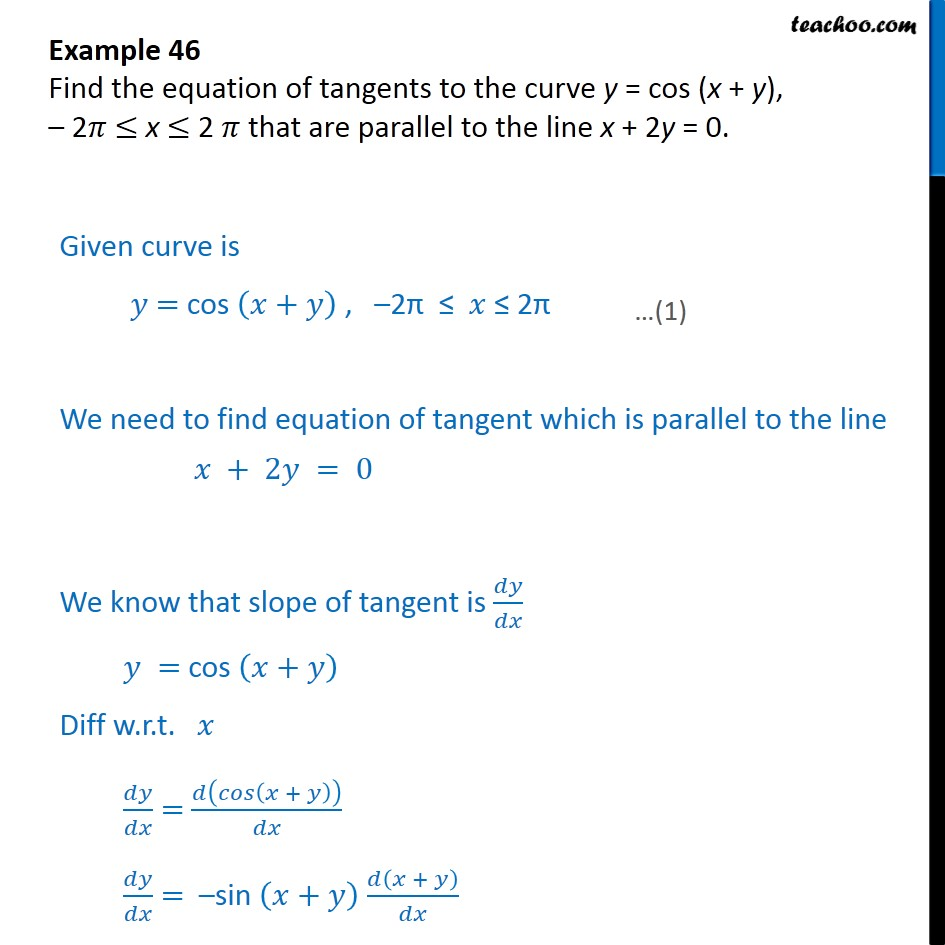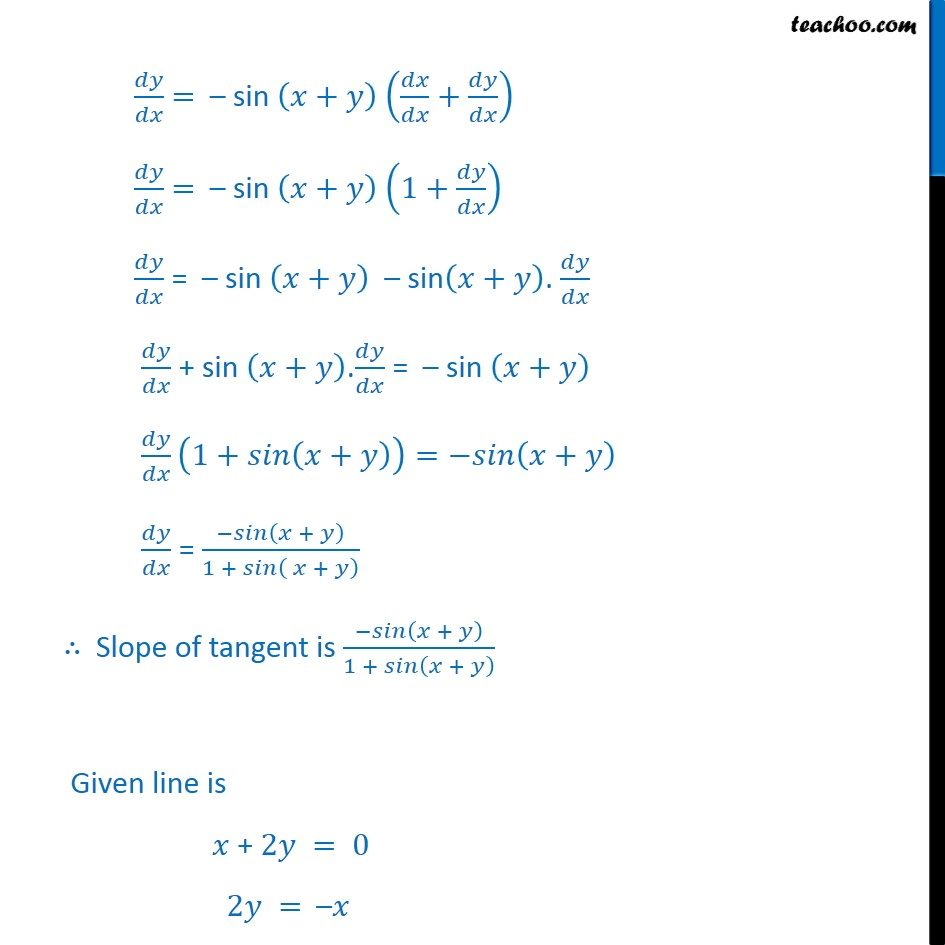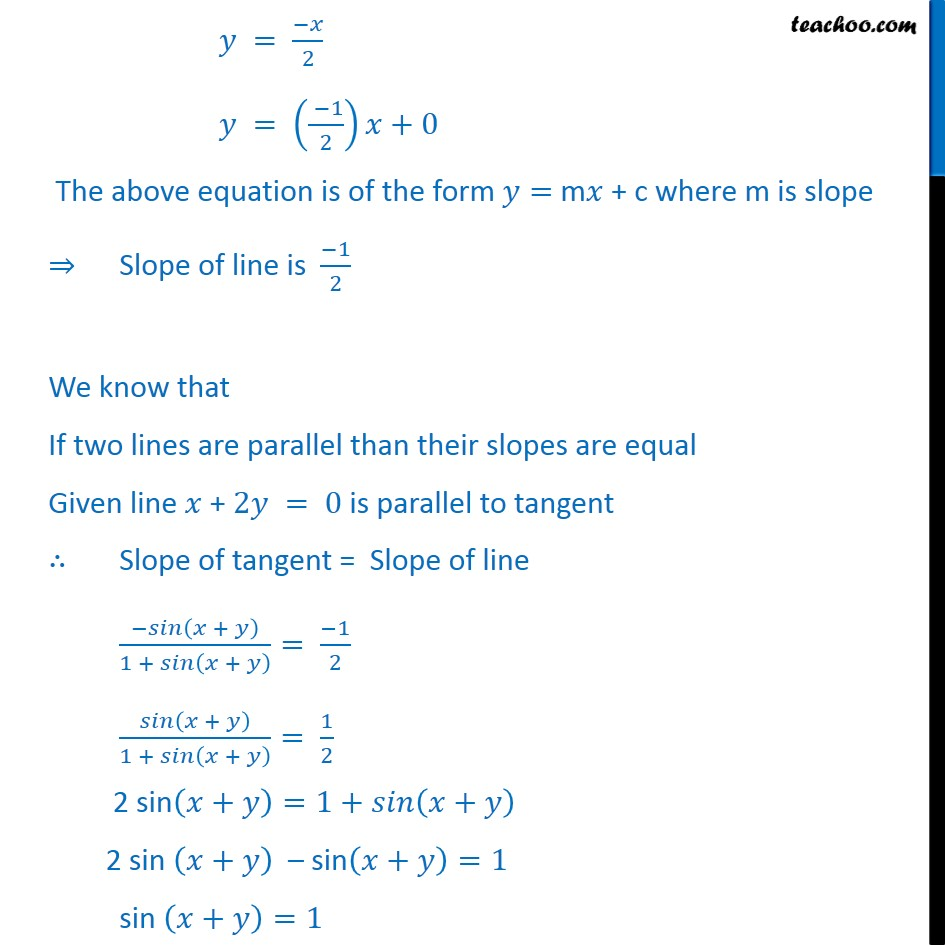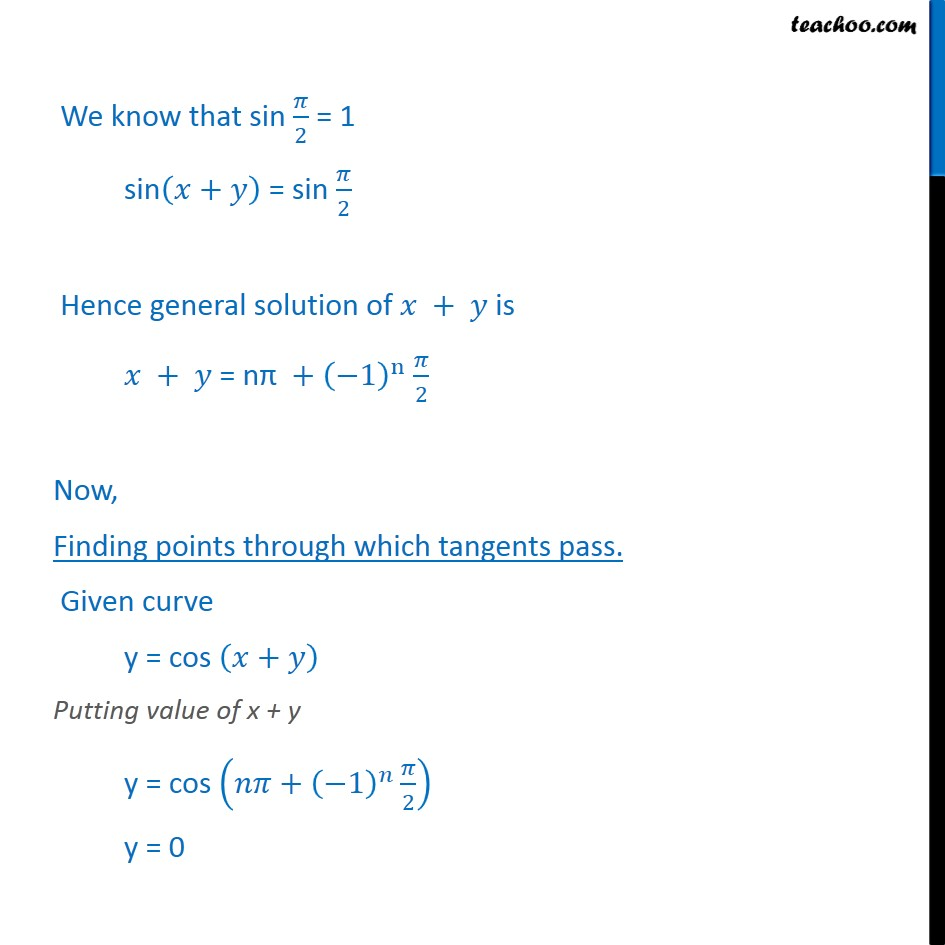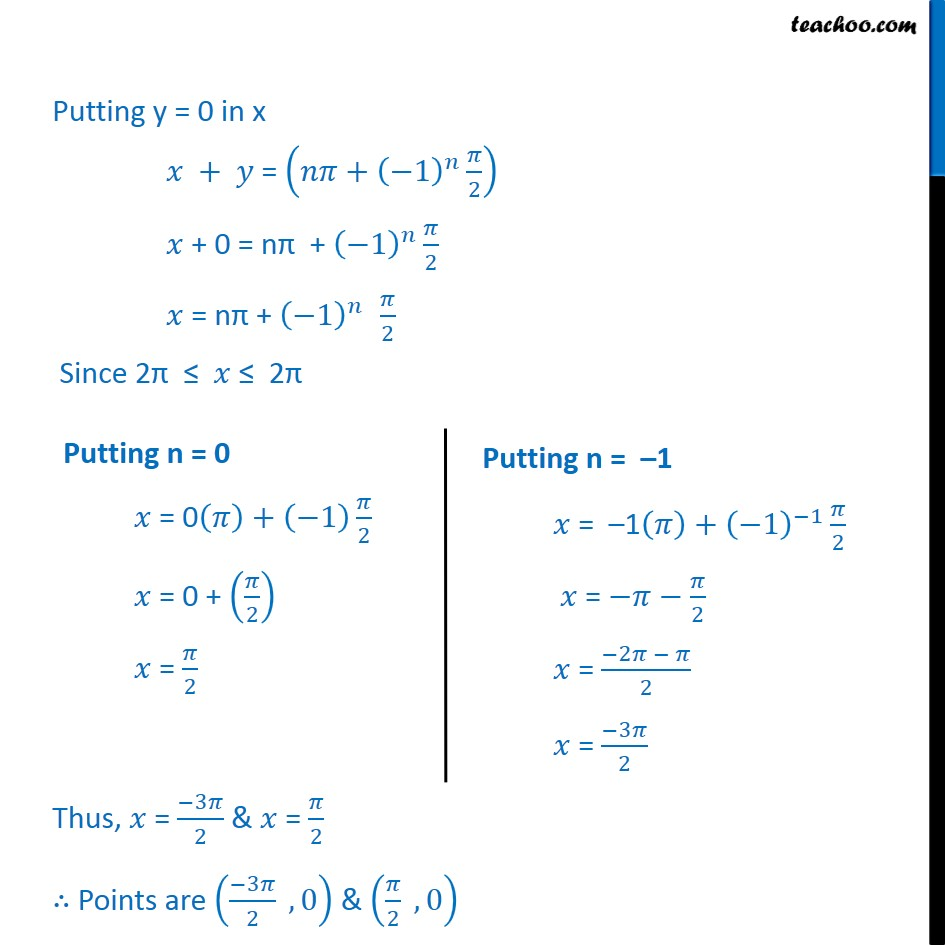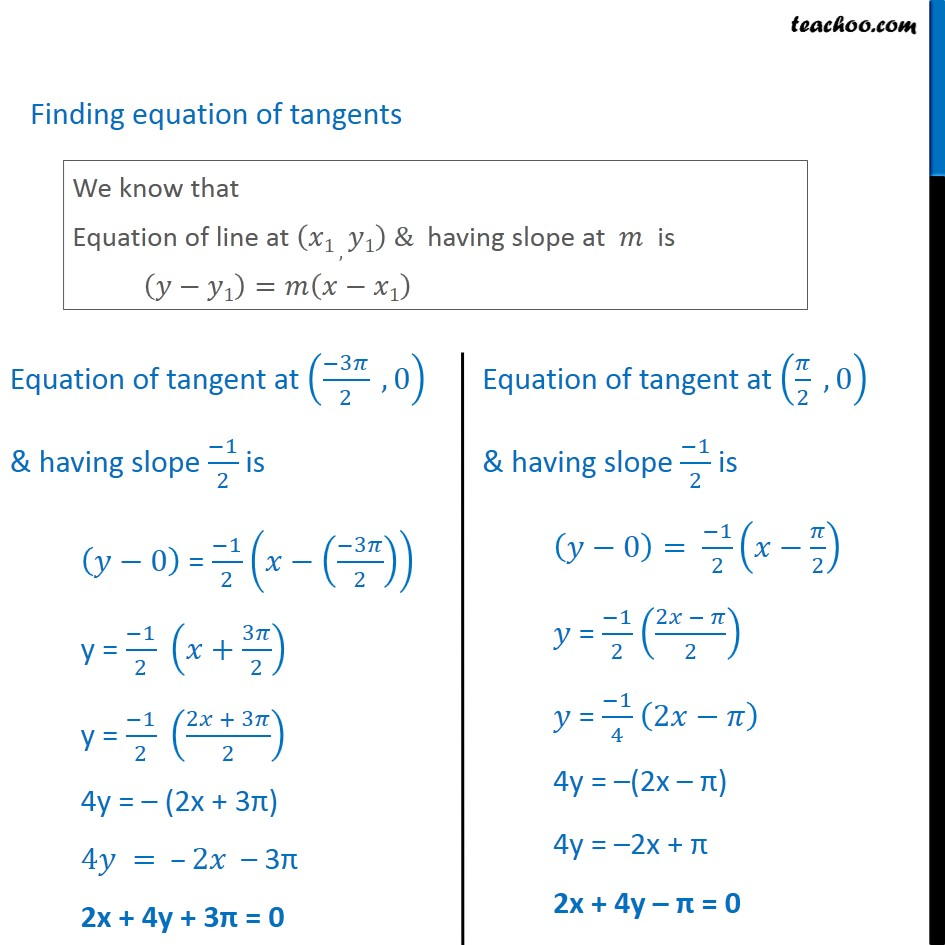1. Chapter 6 Class 12 Application of Derivatives
2. Serial order wise
3. Examples

Transcript

Example 46 Find the equation of tangents to the curve y = cos (x + y), 2 x 2 that are parallel to the line x + 2y = 0. Given curve is = cos + , 2 2 We need to find equation of tangent which is parallel to the line + 2 = 0 We know that slope of tangent is = cos + Diff w.r.t. = + = sin + + = sin + + = sin + 1+ = sin + sin + . + sin + . = sin + 1+ + = + = + 1 + + Slope of tangent is + 1 + + Given line is + 2 = 0 2 = = 2 = 1 2 +0 The above equation is of the form = m + c where m is slope Slope of line is 1 2 We know that If two lines are parallel than their slopes are equal Given line + 2 = 0 is parallel to tangent Slope of tangent = Slope of line + 1 + + = 1 2 + 1 + + = 1 2 2 sin + =1+ + 2 sin + sin + =1 sin + =1 We know that sin 2 = 1 sin + = sin 2 Hence general solution of + is + = n + 1 n 2 Now, Finding points through which tangents pass. Given curve y = cos + Putting value of x + y y = cos + 1 2 y = 0 Putting y = 0 in x + = + 1 2 + 0 = n + 1 2 = n + 1 2 Since 2 2 Thus, = 3 2 & = 2 Points are 3 2 , 0 & 2 , 0 Finding equation of tangents Hence Required Equation of tangent are 2x + 4y + 3 = 0 2x + 4y = 0 Example 46 Find the equation of tangents to the curve y = cos (x + y), 2 x 2 that are parallel to the line x + 2y = 0. Given curve is = cos + , 2 2 We need to find equation of tangent which is parallel to the line + 2 = 0 We know that slope of tangent is = cos + Diff w.r.t. = + = sin + + = sin + + = sin + 1+ = sin + sin + . + sin + . = sin + 1+ + = + = + 1 + + Slope of tangent is + 1 + + Given line is + 2 = 0 2 = = 2 = 1 2 +0 The above equation is of the form = m + c where m is slope Slope of line is 1 2 We know that If two lines are parallel than their slopes are equal Given line + 2 = 0 is parallel to tangent Slope of tangent = Slope of line + 1 + + = 1 2 + 1 + + = 1 2 2 sin + =1+ + 2 sin + sin + =1 sin + =1 We know that sin 2 = 1 sin + = sin 2 Hence general solution of + is + = n + 1 n 2 Now, Finding points through which tangents pass. Given curve y = cos + Putting value of x + y y = cos + 1 2 y = 0 Putting y = 0 in x + = + 1 2 + 0 = n + 1 2 = n + 1 2 Since 2 2 Thus, = 3 2 & = 2 Points are 3 2 , 0 & 2 , 0 Finding equation of tangents Hence Required Equation of tangent are 2x + 4y + 3 = 0 2x + 4y = 0 Example 46 Find the equation of tangents to the curve y = cos (x + y), 2 x 2 that are parallel to the line x + 2y = 0. Given curve is = cos + , 2 2 We need to find equation of tangent which is parallel to the line + 2 = 0 We know that slope of tangent is = cos + Diff w.r.t. = + = sin + + = sin + + = sin + 1+ = sin + sin + . + sin + . = sin + 1+ + = + = + 1 + + Slope of tangent is + 1 + + Given line is + 2 = 0 2 = = 2 = 1 2 +0 The above equation is of the form = m + c where m is slope Slope of line is 1 2 We know that If two lines are parallel than their slopes are equal Given line + 2 = 0 is parallel to tangent Slope of tangent = Slope of line + 1 + + = 1 2 + 1 + + = 1 2 2 sin + =1+ + 2 sin + sin + =1 sin + =1 We know that sin 2 = 1 sin + = sin 2 Hence general solution of + is + = n + 1 n 2 Now, Finding points through which tangents pass. Given curve y = cos + Putting value of x + y y = cos + 1 2 y = 0 Putting y = 0 in x + = + 1 2 + 0 = n + 1 2 = n + 1 2 Since 2 2 Thus, = 3 2 & = 2 Points are 3 2 , 0 & 2 , 0 Finding equation of tangents Hence Required Equation of tangent are 2x + 4y + 3 = 0 2x + 4y = 0

Examples